Wir verwenden Google für unsere Suche. Mit Klick auf „Suche aktivieren“ aktivieren Sie das Suchfeld und akzeptieren die Nutzungsbedingungen.

# New in SG-Lib 2.1

###### exp_2015_02_25- EXPERMENT to create 4x4 flexible hinge strips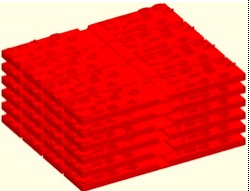% exp_2015_02_25 - EXPERMENT to create 4x4 flexible hinge strips% (by Tim Lueth, VLFL-Lib, 2015-FEB-25 as class: EXPERIMENTS)% % Testing the possibility to create foil hinges by considering Erhard % (2008), page 361ff. The result is written into a binary STL file for % printing.% % LITERATURE:% - Erhard, Gunter (2008): Konstruieren mit Kunststoffen, Carl Hanser % Verlag, München, 4. Auflage% % SG=exp_2015_02_25% === OUTPUT RESULTS ======% SG: Solid geometry of the parts%
###### exp_2015_02_24(s,w,e,x,y)- EXAMPLE how to create contour for a flexible Hinge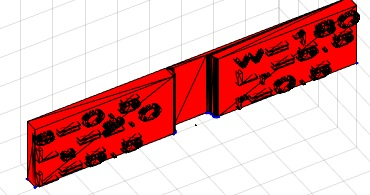% exp_2015_02_24(s,w,e,x,y) - EXAMPLE how to create contour for a % flexible Hinge% (by Tim Lueth, VLFL-Lib, 2015-FEB-24 as class: KINEMATICS AND FRAMES)% % LITERATURE:% - Erhard, Gunter (2008): Konstruieren mit Kunststoffen, Carl Hanser % Verlag, München, 4. Auflage% % [PL,SG]=exp_2015_02_24([s,w,e,x,y])% === INPUT PARAMETERS ===% s: thickness of the hinge% w: radius to bend% e: strain value; default is 40% (0.4)% x: % y: % === OUTPUT RESULTS ======% PL: Point List% SG: Solid Geoemtry%
###### exp_2015_02_23 (r,th,st)- EXPERIMENT Testing perforated PLates by Air pressure and water pressure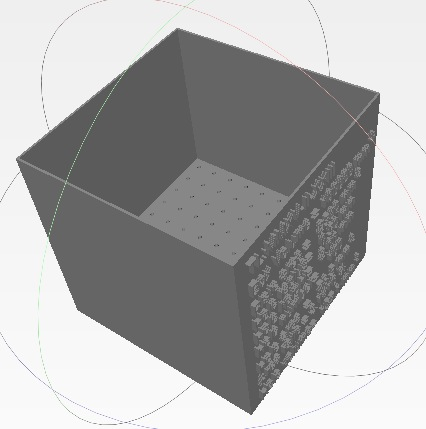% exp_2015_02_23 (r,th,st) - EXPERIMENT Testing perforated PLates by Air % pressure and water pressure% (by Tim Lueth, VLFL-Lib, 2015-FEB-23 as class: MODELING PROCEDURES)% % This box can be filled with water (0.1l) or put ontop of the mouth to % breath trough. During the printing test performed 2015-02-24, it became % clear that it is possible to breath event trough hole with diameter % 0.25mm. (Status of: 2015-02-23)% % exp_2015_02_23([r,th,st])% === INPUT PARAMETERS ===% r: radius of the holes% th: height of the membrane plate% st: step size between the holes%
###### exp_2015_02_22- EXPERIMENT that return rotating shells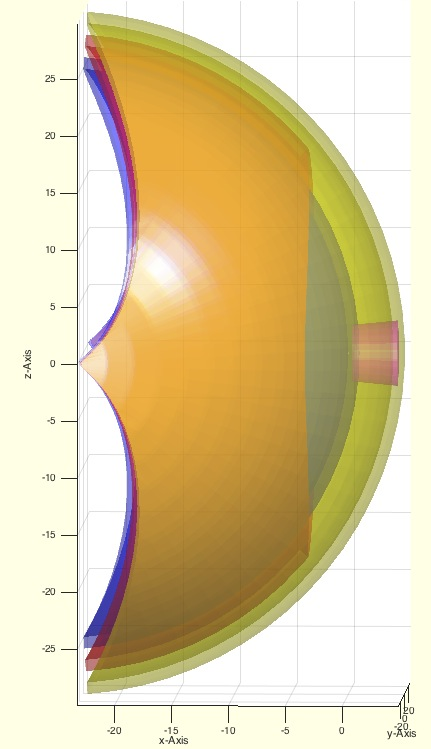% exp_2015_02_22 - EXPERIMENT that return rotating shells% (by Tim Lueth, VLFL-Lib, 2015-FEB-22 as class: MODELING PROCEDURES)% % exp_2015_02_22%
###### SGofCPLsphere(CPL,Ri,d,ns)- returns solid geometry of a wrapped shell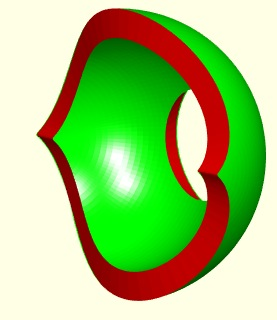% SGofCPLsphere(CPL,Ri,d,ns) - returns solid geometry of a wrapped shell% (by Tim Lueth, VLFL-Lib, 2015-FEB-22 as class: MODELING PROCEDURES)% % See also: SGofCPLsphere, SGbeating, SGofCPLz, SGofCPLT% % SG=SGofCPLsphere(CPL,Ri,d,[ns])% === INPUT PARAMETERS ===% CPL: Closed Polygon List% Ri: Radius of the sphere used for wrapping% d: Thickness/height of the shell% ns: distance between auxiliary points; default is sofrd(Ri)% === OUTPUT RESULTS ======% SG: Solid geometry% % EXAMPLE: Create an wrapped circular patch% SGofCPLshere(CPLofPL({PLcircle(30),PLcircle(10)}),20,0.5)%
###### SGplateunder(SG,h,d)- returns a plate that is completely under the solid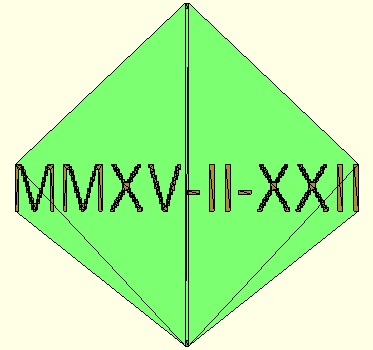% SGplateunder(SG,h,d) - returns a plate that is completely under the % solid% (by Tim Lueth, VLFL-Lib, 2015-FEB-22 as class: MODELING PROCEDURES)% % plate shape is a parallel projection of the outer contour onto the x/y % plane. This procedure is used by VLFLimageoftext. (Status of: % 2015-02-22)% % SGP=SGplateunder(SG,[h,d])% === INPUT PARAMETERS ===% SG: Solid Geometry% h: height of the plate; default is 1% d: gap between solid and plate (can be negative)% === OUTPUT RESULTS ======% SGP: Plate under SG%
###### exp_2015_02_21 (R,stext,H,n)- creates a honory medallie% exp_2015_02_21 (R,stext,H,n) - creates a honory medallie% (by Tim Lueth, VLFL-Lib, 2015-FEB-21 as class: MODELING PROCEDURES)% % exp_2015_02_21([R,stext,H,n])% === INPUT PARAMETERS ===% R: Radius; default is 35% stext: Text; dafault is todays date% H: Height; default is R/10% n: number of edges; default is circle%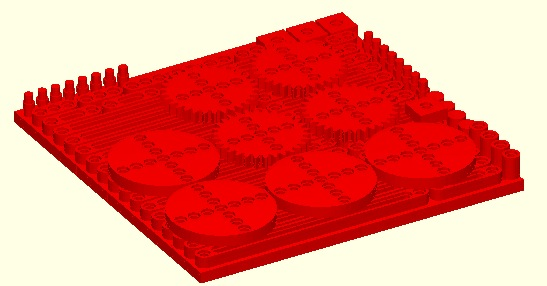% fourBarLinkageKit(task,2,3) - Creation kit for Four-Bar-Linkages% (by Tim Lueth, VLFL-Lib, 2015-FEB-20 as class: KINEMATICS AND FRAMES)% % Depending on the input parameter this procedures returns parts of a % creation kit or a complete creation kit for physical experiments with % 4-Bar-linkages, gears etc:% It is similar to exp_2015_02_19 but with a more primitive modeler.% 'Knob': Knob for turning parts manually or as stopper% 'Bar': Basic elements of a linkage% 'Spacer': spacing element required for some configurations% 'Bolt': turning bolt as axis to connect two bars as joint% 'Shaft': shaft for fixed connection between two bars/parts% 'Wheel': Turning wheel with sockets for shafts and bolts% 'Gear': Turning gear (m=1) with sockets for shafts and bolts% 'Brick': Basic element with sockets for shafts and bolts% 'Plate': Square grid of bricks as base plate for experiments% 'Test': Small plate plus limited numbers of bars for test the 3D-Printer% 'all': All parts for a 4-Bar-Linkage construction kit% 'kit': Same as all but including a box/case% % (Status of: 2015-02-20)% % SG=fourBarLinkageKit([task,2,3])% === INPUT PARAMETERS ===% task: String for Task, default is bar% 2: 2nd parameter for shapes% 3: 3rn parameter for shapes/mode% === OUTPUT RESULTS ======% SG: % % EXAMPLE: % fourBarLinkageKit ('gear')% fourBarLinkageKit ('all')% fourBarLinkageKit ('kit')% %
###### SGstretching(A,dx,xlim)- cuts a solid geometry in x and stretches in x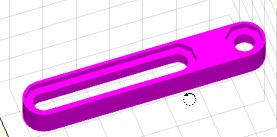% SGstretching(A,dx,xlim) - cuts a solid geometry in x and stretches in x% (by Tim Lueth, VLFL-Lib, 2015-FEB-20 as class: SURFACES)% % A=SGstretching(A,dx,xlim)% === INPUT PARAMETERS ===% A: Solid geometry% dx: add d to all x-coodinates% xlim: for all x-coordinates > xlim% === OUTPUT RESULTS ======% A: Stretched solid geoemtry%
###### SGstripfields(SG)- remove unneccessary fields in SG structs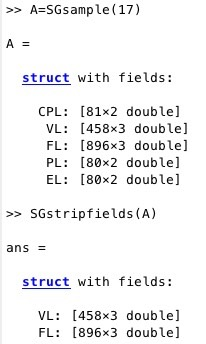% SGstripfields(SG) - remove unneccessary fields in SG structs% (by Tim Lueth, VLFL-Lib, 2015-FEB-20 as class: AUXILIARY PROCEDURES)% % removes CPL,PL,EL (Status of: 2017-03-20)% % See also: SGsample% % SG=SGstripfields(SG)% === INPUT PARAMETERS ===% SG: Solid Geoemtry% === OUTPUT RESULTS ======% SG: Solid Geoemtry%
###### VLplotmotion(VL,i)- draws position, speed and acceleration of a VL% VLplotmotion(VL,i) - draws position, speed and acceleration of a VL% (by Tim Lueth, VLFL-Lib, 2015-FEB-16 as class: KINEMATICS AND FRAMES)% % VLplotmotion(VL,[i])% === INPUT PARAMETERS ===% VL: Vertex list% i: optional angle list%
###### exp_2015_02_14- Experiment that shows the symbolic equation solution of a 4-Bar-Linkage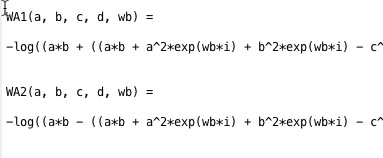% exp_2015_02_14 - Experiment that shows the symbolic equation solution of a 4-Bar-Linkage% (by Tim Lueth, VLFL-Lib, 2015-FEB-13 as class: EXPERIMENTS)% % This Matlab script/fnctn shows how the analytical solution for the % forward kinematics of a Four-Bar-Linkage can be calcuated. Typically % the final result is used later for numerical fnctn, i.e. the calculated % symbolic equation is used as code line in a fnctn such as % 'fourBarLinkage' (Status of: 2015-02-18)% % Introduced first in SolidGeometry 2.1% % See also: fourBarLinkage% % exp_2015_02_14% % See also: fourBarLinkage% % % Copyright 2015-2018 Tim C. Lueth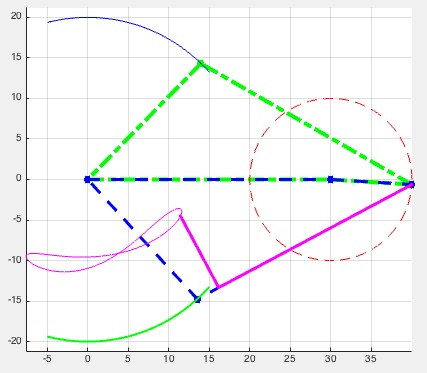% fourBarLinkage(a,b,c,d,wb,k1,k2) - multi purpose fnctn for a 4-Bar-Linkage% (by Tim Lueth, VLFL-Lib, 2015-FEB-13 as class: KINEMATICS AND FRAMES)% % The core of this fnctn are the two analytical solutions for the % calculation of the closed 4-Bar-linkage angle. The fnctn calculates for % given link length a, b, c, d and an angle for phi-b, the two solutions % for closing the linkage and returns the solution points% without an output parameter the configuration is plotted.% without input parameter wb, a drawing is created to explain the linkage% Gestell = Ground% Kurbel = Crank% Koppel = Coupler% Schwinge = Follower% (Status of: 2018-06-02)% % Introduced first in SolidGeometry 2.1% % See also: exp_2015_02_14, exp_2013_06_15, exp_2013_06_16, fourbarlinkage% % [C,s,D1,D2,wb,wa1,wa2]=fourBarLinkage(a,b,c,d,[wb,k1,k2])% === INPUT PARAMETERS ===% a: ground distance; alternative Point 1% b: crank length; alternative Point 2% c: coupler length; alternative Point 3% d: Follower length; alternative Point 4% wb: rotation angle at a/b% k1: C+k1*(D1-C)% k2: orthogonal to k1 in mm% === OUTPUT RESULTS ======% C: Point C% s: solution exist or not% D1: Point D1% D2: Point D2% wb: rotation angle at a/b% wa1: rotation angle at a/d (solution 1)% wa2: rotation angle at a/d (solution 2)% % EXAMPLE: To see the use simply try% fourBarLinkage (30,40,30,20);% fourBarLinkage (30,40,30,20,[],0.5);% fourBarLinkage (30,10,30,20,[],0.5);% % See also: exp_2015_02_14, exp_2013_06_15, exp_2013_06_16, fourbarlinkage% % % Copyright 2015-2018 Tim C. Lueth
###### exp_2015_02_13- shows the symbolic equation solution for a three bar linkage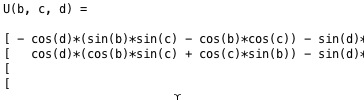% exp_2015_02_13 - shows the symbolic equation solution for a three bar % linkage% (by Tim Lueth, , 2015-FEB-13 as class: EXPERIMENTS)% % Mainly for educational use!% (Status of: 2015-02-18)% % exp_2015_02_13%
###### KMchain(KM,chain)- calculates a kinematic structure from a given frame chain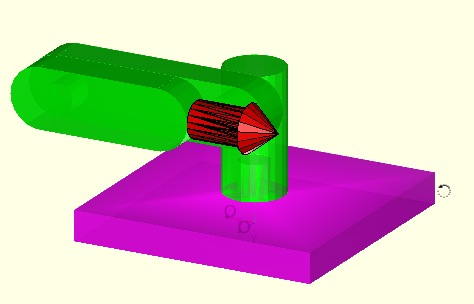% KMchain(KM,chain) - calculates a kinematic structure from a given frame % chain% (by Tim Lueth, VLFL-Lib, 2015-FEB-10 as class: KINEMATICS AND FRAMES)% % KM=KMchain(KM,chain)% === INPUT PARAMETERS ===% KM: Kinematic model {SG,'Name',HT-Matrix}% chain: string 'A.A-A.B-' % === OUTPUT RESULTS ======% KM: Kinematic model with adjusted Frames KM{:,3}% % EXAMPLE: Show a robot example:% %
###### KMplot (KM)- plots all parts of a kinematic model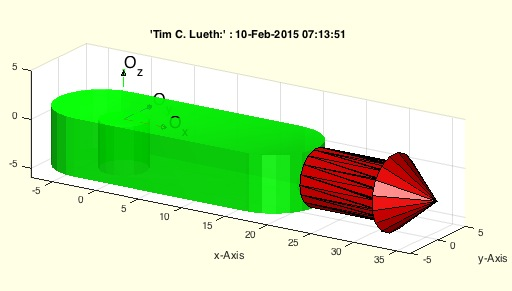% KMplot (KM) - plots all parts of a kinematic model% (by Tim Lueth, VLFL-Lib, 2015-FEB-10 as class: KINEMATICS AND FRAMES)% % A kinematic model is a cell list {nx3} of the format {, % , 4x4-Matrix}. The Solid geometry is a struct consisting of % vertex list (VL) and facet list (FL) and a cell list of 4x4 % Frame-matrices (T) and a cell list of Frame-name-strings (Tname). % (Status of: 2015-02-10)% % KMplot(KM)% === INPUT PARAMETERS ===% KM: Kinematic model%
###### KMT(KM,N);- returns a HT-Matrix from a kinematic model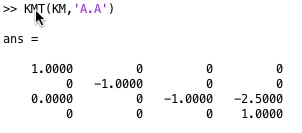% KMT(KM,N); - returns a HT-Matrix from a kinematic model% (by Tim Lueth, VLFL-Lib, 2015-FEB-09 as class: KINEMATICS AND FRAMES)% % A kinematik model currently consists of a list of solids an a list of % strings that can be used to identify the solid in the list.% An error occurs if either the solid name is not existing in the model % or the frame (Status of: 2015-02-09)% % T=KMT(KM,N);% === INPUT PARAMETERS ===% KM: Kinematic Model {Solid ' Name'}% N: Name "Solid.Frame"% === OUTPUT RESULTS ======% T: HT Matrix%
###### exp_2015_02_09- EXPERIMENT to show a serial kinematic chain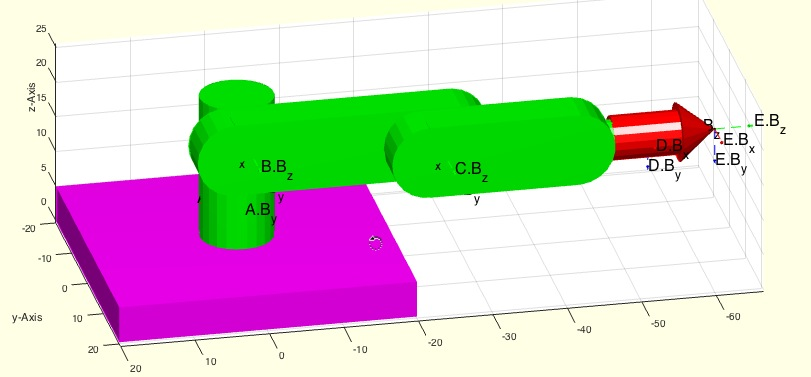% exp_2015_02_09 - EXPERIMENT to show a serial kinematic chain% (by Tim Lueth, VLFL-Lib, 2015-FEB-09 as class: EXPERIMENTS)% % exp_2015_02_09%
###### SGT(SGN,N,SN)- returns a called HT-Frame from a solid's struct% SGT(SGN,N,SN) - returns a called HT-Frame from a solid's struct% (by Tim Lueth, VLFL-Lib, 2015-FEB-03 as class: KINEMATICS AND FRAMES)% % =======================================================================% OBSOLETE (2018-07-29) - USE 'SGTget, SGTplot' INSTEAD% =======================================================================% % Will be removed in future release. Use SGTget instead% if no output parameter is specified, the solid is plotted including the % frames (Status of: 2018-07-29)% % Introduced first in SolidGeometry 2.1% % See also: [ SGTget, SGTplot ] ; SGTget, SGTset, SGTplot, SGTremove, % SGTui% % [T,a]=SGT(SGN,[N,SN])% === INPUT PARAMETERS ===% SGN: Solid Geoemtry (VL,FL,TName,T)% N: String% SN: Name of Solid Geoemtry for plotting% === OUTPUT RESULTS ======% T: Transformation matrix% a: index if Frame in Frame cells list% % EXAMPLE: % loadweb JACO_robot.mat;% SGfigure; view(-30,30); SGTplot(JC0,'','A')% % See also: [ SGTget, SGTplot ] ; SGTget, SGTset, SGTplot, SGTremove, % SGTui% % % Copyright 2015-2018 Tim C. Lueth
###### SGTremove(SG,N)- removes a transformation frame from a solid geometry% SGTremove(SG,N) - removes a transformation frame from a solid geometry% (by Tim Lueth, VLFL-Lib, 2015-FEB-01 as class: KINEMATICS AND FRAMES)% % to reduce problems with wrong parameter calls, only the name 'all' % should remove all frames of the solid.% Anyway, currently also the call without any name parameter (nargin==1) % will remove all frames; (Status of: 2017-04-18)% % Introduced first in SolidGeometry 2.1% % See also: SGTget, SGTset, SGTplot, SGTui% % SG=SGTremove(SG,N)% === INPUT PARAMETERS ===% SG: SG (VL/FL plus Tname, T, TFiL)% N: string with name of Frame; 'all' removes all frames% === OUTPUT RESULTS ======% SG: SG without named frame% % EXAMPLE: Set and remove a frame% SG=SGTui(SGbox([30 20 10])), SGTremove(SG,'A')% % See also: SGTget, SGTset, SGTplot, SGTui% % % Copyright 2015-2017 Tim C. Lueth
###### SGTui(SG,N,fe,FS,Rz)- returns a HT matrix for a manuel selected union space% SGTui(SG,N,fe,FS,Rz) - returns a HT matrix for a manuel selected union space% (by Tim Lueth, VLFL-Lib, 2015-FEB-01 as class: KINEMATICS AND FRAMES)% % This is a user interface for manually selecting union patches or tips % (vertices) to generate frames for linking HT matrices to the solid % geometries. It support 3 types of frames% a) tips% b) planar surfaces% c) spherical surfaces with feature edges% SGT changed in SG-Lib 3.9 and supports also centers of circular borders% (Status of: 2017-07-21)% % Introduced first in SolidGeometry 2.1% % See also: SGTget, SGTset, SGTplot, SGTremove, VLFLsurfaceofSGT% % [SGN,T,h,Rorg]=SGTui(SG,[N,fe,FS,Rz])% === INPUT PARAMETERS ===% SG: Solid Geometry% N: Name of the new/replaced Frame % fe: feature edge angle; default 0.08; use 1 for freeform surfaces% FS: Optional string selector for center points such as 'R1'% Rz: Optional Rz rotation angle% === OUTPUT RESULTS ======% SGN: New SG with Tname, T, TFiL% T: Transformation matrix% h: handle to graphic% Rorg: % % EXAMPLE: load AIM_SGrobot; SG3=SGTremove(SG3,'all');% SG3=SGTui(SG3,[],0.3)% % See also: SGTget, SGTset, SGTplot, SGTremove, VLFLsurfaceofSGT% % % Copyright 2015-2017 Tim C. Lueth
###### SGmelting(SG)- returns a surface model without intrusion of solids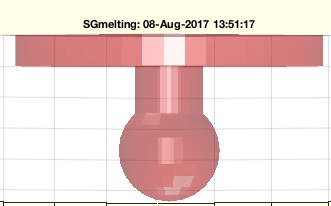% SGmelting(SG) - returns a surface model without intrusion of solids% (by Tim Lueth, VLFL-Lib, 2015-FEB-01 as class: 3D MANUFACTURING)% % =======================================================================% OBSOLETE (2017-08-08) - USE FAMILY 'SGsurfacemeltbool' INSTEAD% =======================================================================% % This fnctn is necessary if slicer programs as part of the 3D % manufacturing process are unable to handle intrusions of separated % surface models within one STl-File.% It is slow since it uses intensively SGbool (Status of: 2015-02-11)% % Introduced first in SolidGeometry 2.1% % See also: [ SGsurfacemeltbool ] ; SGbool% % SGN=SGmelting(SG)% === INPUT PARAMETERS ===% SG: Surface model that contains separated surfaces% === OUTPUT RESULTS ======% SGN: % % See also: [ SGsurfacemeltbool ] ; SGbool% % % Copyright 2015-2017 Tim C. Lueth
###### TR3mountingfaces(TR3,fi,fe)- finds connected facets with same normal vector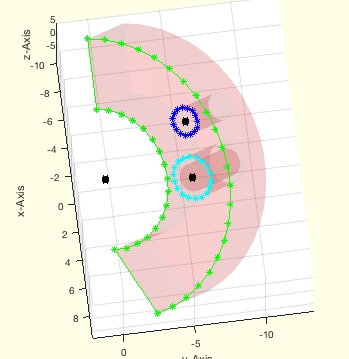% TR3mountingfaces(TR3,fi,fe) - finds connected facets with same normal vector% (by Tim Lueth, VLFL-Lib, 2015-FEB-01 as class: SURFACES)% % Powerful but slow/recursive fnctn to detect points that defined planar % and spherical surfaces. % Auxiliary fnctn developed originally for and used in SGTui (Status of: % 2018-08-01)% % Introduced first in SolidGeometry 2.1% % See also: TR3mountingfaces, MLofSG, SGTui% % [FIL,nv,FnL,NnL,RL,CVL,TR3]=TR3mountingfaces(TR3,fi,[fe])% === INPUT PARAMETERS ===% TR3: Surface triangulation of a solid% fi: singe facet index% fe: feature edge angle; default is 0% === OUTPUT RESULTS ======% FIL: all facets connected to first facet with the same normal vector% nv: normal vector of this facet% FnL: all neigbor facets of FIL. WARNING: Always empty if fe>0% NnL: normal vector angle difference to nv of FnL% RL: Radial list created by CVLdimclassifier% CVL: CLosed Vertex list used for CVLdimclassifier% TR3: TR# is case that an SG was used as input parameter% % EXAMPLE: % SG=SGtransR(SGsample(25),rot(pi/6,0,pi/2)); % TR3=triangulation(SG.FL,SG.VL);% [a,b,c,d,RL,CVL]=TR3mountingfaces(TR3,60,1);% SGfigure; h=SGplot(SG); setplotlight(h,'r',0.1); CVLplot(CVL); % VLplot(RL(:,1:3),'k*',5);% % See also: TR3mountingfaces, MLofSG, SGTui% % % Copyright 2015-2018 Tim C. Lueth
###### SGpacking(SGC,dim,dist,ez,turn)- returns a package of all solids of a cell list% SGpacking(SGC,dim,dist,ez,turn) - returns a package of all solids of a cell list% (by Tim Lueth, VLFL-Lib, 2015-JAN-31 as class: 3D MANUFACTURING)% % arranges the solids in a cell list of solids in a specified volume % (Status of: 2018-08-01)% % Introduced first in SolidGeometry 2.1% % See also: SGsurfaces, SGanalyzeGroupParts, SGboxing, SGboxpacking, % SGpatternXYZ, SGcopyrotZ, SGarrangeSG, SGarrangeSGC, SGCaddSGn, SGCaddSG% % [SGN,SG]=SGpacking(SGC,[dim,dist,ez,turn])% === INPUT PARAMETERS ===% SGC: Cell list of solid geometries% dim: dimension of package, default is [85 65 100];% dist: minimal distance between solids; default is 1;% ez: optional ez vector for turning limitation; default is empty;% turn: true== turn to flat; false==unchanged orientation; default is true% === OUTPUT RESULTS ======% SGN: Packed solid geometry as one solid% SG: Packed solid geometry as cell list% % EXAMPLE: % loadweb JACO_robot.mat; SGpacking(JACO,[600 500 500],10,'',false)% loadweb JACO_robot.mat; SGpacking(JACO,[600 500 500],10,'',true)% % See also: SGsurfaces, SGanalyzeGroupParts, SGboxing, SGboxpacking, % SGpatternXYZ, SGcopyrotZ, SGarrangeSG, SGarrangeSGC, SGCaddSGn, SGCaddSG% % % Copyright 2015-2018 Tim C. Lueth
###### binpacking3D(LAY,BOXL,grid)- returns a straight forward bin packing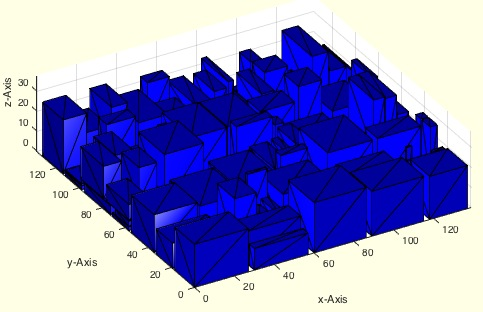% binpacking3D(LAY,BOXL,grid) - returns a straight forward bin packing% (by Tim Lueth, VLFL-Lib, 2015-JAN-31 as class: AUXILIARY PROCEDURES)% % h/2 is a good estimation for a layout size [h/2 h/2 300] if object % height differs% (Status of: 2015-01-31)% % [BOXL,h]=binpacking3D([LAY,BOXL,grid])% === INPUT PARAMETERS ===% LAY: Layout dimensions; default [100,60,80]% BOXL: nx4 [index size-x size-y size-z]% grid: grid size; default is 1 mm% === OUTPUT RESULTS ======% BOXL: nx4 [index pos-x pos-y pos-z]% h: estimation of a square side length if all bin have the same heigth% % EXAMPLE: Just enter: binpacking3D%
###### PLtransT(PL,T)- rotates and moves a point list nx2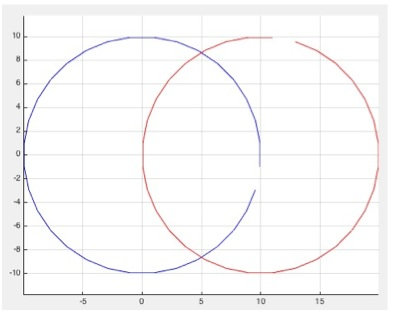% PLtransT(PL,T) - rotates and moves a point list nx2% (by Tim Lueth, VLFL-Lib, 2015-JAN-30 as class: ANALYTICAL GEOMETRY)% % Introduced first in SolidGeometry 2.1% % See also: PLtransP, PLtransR, PLtrans, PLtrans0, PLtrans1, PLtransC% % PL=PLtransT(PL,T)% === INPUT PARAMETERS ===% PL: Point list nx2% T: Transformation matrix 2x3 or 3x3% === OUTPUT RESULTS ======% PL: Point list nx2% % See also: PLtransP, PLtransR, PLtrans, PLtrans0, PLtrans1, PLtransC% % % Copyright 2015-2018 Tim C. Lueth
###### PLtrans1(PL)- returns a point list moved into the first quadrant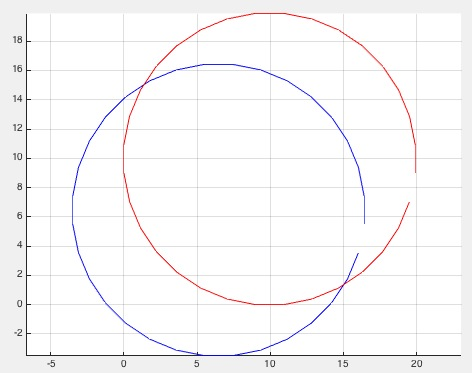% PLtrans1(PL) - returns a point list moved into the first quadrant% (by Tim Lueth, VLFL-Lib, 2015-JAN-30 as class: ANALYTICAL GEOMETRY)% % Introduced first in SolidGeometry 2.1% % See also: PLtransP, PLtransR, PLtrans, PLtrans0, PLtransT, PLtransC% % PL=PLtrans1(PL)% === INPUT PARAMETERS ===% PL: Point list nx2% === OUTPUT RESULTS ======% PL: Point list nx2% % See also: PLtransP, PLtransR, PLtrans, PLtrans0, PLtransT, PLtransC% % % Copyright 2015-2018 Tim C. Lueth
###### PLtrans0(PL)- returns an origin centered point list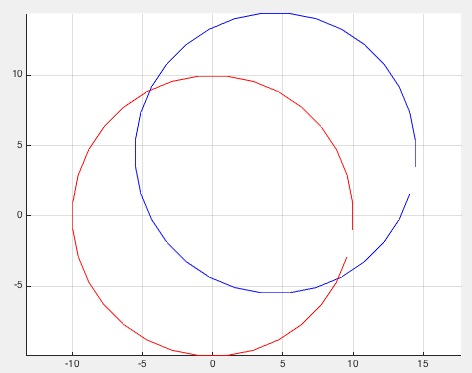% PLtrans0(PL) - returns an origin centered point list% (by Tim Lueth, VLFL-Lib, 2015-JAN-30 as class: AUXILIARY PROCEDURES)% % Introduced first in SolidGeometry 2.1% % See also: PLtransP, PLtransR, PLtrans, PLtrans1, PLtransT, PLtransC% % PL=PLtrans0(PL)% === INPUT PARAMETERS ===% PL: Point list nx2% === OUTPUT RESULTS ======% PL: Point list nx2% % See also: PLtransP, PLtransR, PLtrans, PLtrans1, PLtransT, PLtransC% % % Copyright 2015-2018 Tim C. Lueth
###### PLtrans(PLin,T)- multi transformation modes for a point list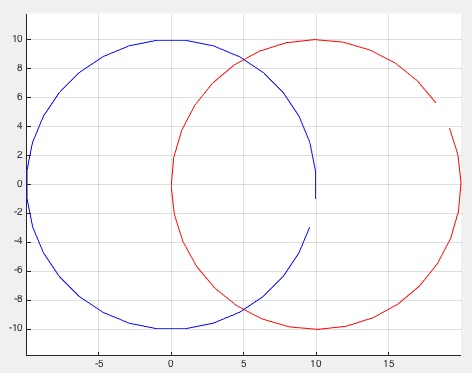% PLtrans(PLin,T) - multi transformation modes for a point list% (by Tim Lueth, VLFL-Lib, 2015-JAN-30 as class: ANALYTICAL GEOMETRY)% % Does currently not support a cells of points list% Combines PLtrans0, PLtrans1, PLtransP, PLtransR, PLtransT depending on % the transformation value% % (Status of: 2018-07-31)% % Introduced first in SolidGeometry 2.1% % See also: PLtransP, PLtransR, PLtrans0, PLtrans1, PLtransT, PLtransC% % [PLout,varargout]=PLtrans(PLin,T)% === INPUT PARAMETERS ===% PLin: Point list nx2% T: Transformation Value, Vector, or Matrix% === OUTPUT RESULTS ======% [Lout,varargout]=PLtrans(PLin,T)% % if size(T,1)==1 && size(T,2)==1% if T==0]: Point list nx2% [Lout,varargout]=PLtrans(PLin,T)% % if size(T,1)==1 && size(T,2)==1% if T==0]: not used yet% % EXAMPLE: try:% PLtrans(PLcircle(10),[rotdeg(40) [10;0]])% PLtrans(rand(20,2),0)% PLtrans(rand(20,2),1)% PLtrans(rand(20,2),[10, 0])% PLtrans(rand(20,2),[10; 0])% PLtrans(rand(20,2),rot(pi/3))% PLtrans(rand(20,2),rotdeg(40))% PLtrans(rand(20,2),[rotdeg(40) [10;0]])% % See also: PLtransP, PLtransR, PLtrans0, PLtrans1, PLtransT, PLtransC% % % Copyright 2015-2018 Tim C. Lueth
###### AIM_Robot- returns a solid geometry for a robot used in AIM lectures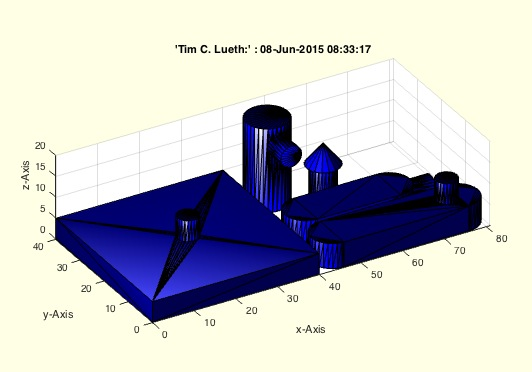% AIM_Robot - returns a solid geometry for a robot used in AIM lectures% (by Tim Lueth, VLFL-Lib, 2015-JAN-30 as class: MODELING PROCEDURES)% % exatly the same as exp_2015_01_30 (Status of: 2015-06-08)% % SGrobot=AIM_Robot% === OUTPUT RESULTS ======% SGrobot: Solid Geometry of a printable Robot for AIM%
###### exp_2015_01_30- returns a solid geometry for a robot used in AIM lectures% exp_2015_01_30 - returns a solid geometry for a robot used in AIM % lectures% (by Tim Lueth, VLFL-Lib, 2015-JAN-30 as class: MODELING PROCEDURES)% % SGrobot=exp_2015_01_30% === OUTPUT RESULTS ======% SGrobot: Solid Geometry of a printable Robot for AIM%
###### gets(allargins,paramstr,tab)- processes automatically the varargins of a function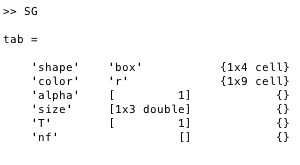###### T2plot(p,c,w,N,N)- plots a HT matrix as straight line vector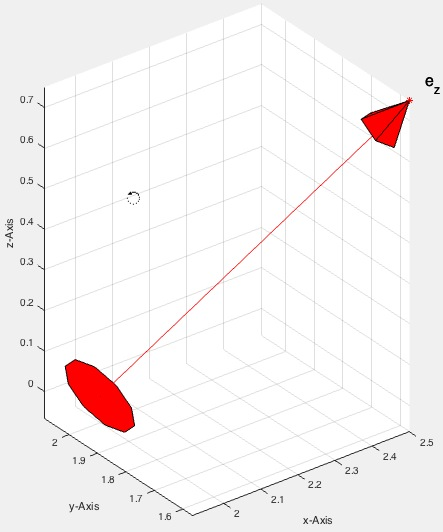% T2plot(p,c,w,N,N) - plots a HT matrix as straight line vector% (by Tim Lueth, VLFL-Lib, 2015-JAN-27 as class: VISUALIZATION)% % See also: pplot, lplot, tfplot, tlplot, slplot, plotTP, plotL, plotT% % T2plot(p,[c,w,N,N])% === INPUT PARAMETERS ===% p: Point or % c: color% w: width% N: String for name% N: %
###### slplot (p,ez,c,w,l,d)- straight line plot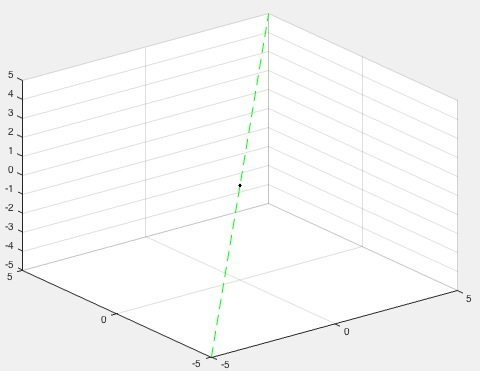% slplot (p,ez,c,w,l,d) - straight line plot% (by Tim Lueth, VLFL-Lib, 2015-JAN-26 as class: VISUALIZATION)% % See also: getvar, pplot, lplot, tfplot, tlplot, plotTP, plotL, plotT, % T2Plot% % slplot(p,[ez,c,w,l,d])% === INPUT PARAMETERS ===% p: starting point% ez: direction if straight line% c: line color% w: line width; default is 1% l: line length; default is 10% d: color of the starting point% === PROPERTY NAMES =====% 'color' : Color of the straight line; default is 'r--'% 'point' : Color of the start point; default is 'k.'% 'length' : Length of the line; default is 10% 'lineWidth' : Width of the straight line; default is 1% 'color' : % 'point' : % 'length' : % 'lineWidth' : % % EXAMPLE: Plot a green straight line% slplot([0 0 0],[1 1 1],'color','g--')%
###### VMpseudo3D(V,lim,maxc,s)- returns an 2D image of pseudo 3D rendered surface% VMpseudo3D(V,lim,maxc,s) - returns an 2D image of pseudo 3D rendered surface% (by Tim Lueth, VLFL-Lib, 2015-JAN-26 as class: VOXELS)% % Use matlab fnctns permute and flip and view to produce the desired % output (Status of: 2017-02-16)% % See also: VM, VMimage, VMimrot90, VMmontage, VMplot, VMreaddicom, % VMreaddicomdir, VMuidicom% % I=VMpseudo3D(V,lim,[maxc,s])% === INPUT PARAMETERS ===% V: Voxel model% lim: surface intensity value% maxc: maximum used color; default is 4095% s: cut value: start to display the real slice values; default is 0% === OUTPUT RESULTS ======% I: Image% % EXAMPLE: % I=VMpseudo3D(permute(V,[2 3 1]),1600,4095,100); % imshow(I,[0 4095]); axis square, view (-90,90); show% close all; VMpseudo3D(flip(permute(V,[3 2 1]),1),1400,'',200);%
###### VMmontage(V)- plots all images of an image stack into one figure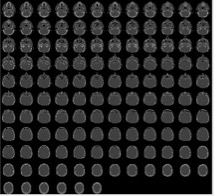% VMmontage(V) - plots all images of an image stack into one figure% (by Tim Lueth, VLFL-Lib, 2015-JAN-24 as class: VOXELS)% % Based on Matlab fnctn montage (Status of: 2017-04-02)% % See also: VMimage, VMintensityscale, VMresize, VMimrot90, VMplot, % VMpseudo3D, VMreaddicom, VMreaddicomdir, VMuidicom% % h=VMmontage(V)% === INPUT PARAMETERS ===% V: Voxel model% === OUTPUT RESULTS ======% h: handle of image%
###### VLscatter(VL,C,n)- plots a vertex list with a corresponding image intensity list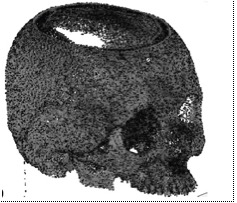% VLscatter(VL,C,n) - plots a vertex list with a corresponding image intensity list% (by Tim Lueth, VLFL-Lib, 2015-JAN-22 as class: VOXELS)% % See also: VLplot, VLofVM% % h=VLscatter(VL,C,n)% === INPUT PARAMETERS ===% VL: Vertex list% C: Intensity List (Hounsfield)% n: step size for vertex selection% === OUTPUT RESULTS ======% h: handle to plot% % EXAMPLE: Scatter a Voxelmodel% VLscatter(VLofVM(V>1600),V(find(V>1600)),10);%
###### VLFLofVMmarchcube(V,vs)- returns a surface model by the marching cubes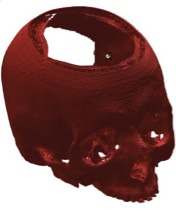% VLFLofVMmarchcube(V,vs) - returns a surface model by the marching cubes% (by Tim Lueth, VLFL-Lib, 2015-JAN-22 as class: VOXELS)% % This fnctn uses the MarchingCubes fnctn from Matlab Central written by % Peter Hammer in 2011 based on the Octave fnctn written by Martin Helm % (www.mhelm.de/octave/m/marching_cube.m) in 2009. It contains also code % from Oliver Woodford for removing duplicated vertices. (Status of: % 2017-02-16)% % See also: VLFLofVMdelaunay% % [VL,FL]=VLFLofVMmarchcube(V,[vs])% === INPUT PARAMETERS ===% V: Logical Volume Model% vs: voxel size, optional% === OUTPUT RESULTS ======% VL: Vertex list% FL: Facet list% % EXAMPLE: [V,vs]=VMreaddicomdir('AIM_DICOMFILES');% [VL,FL]=VLFLofVMmarchcube((V>400)&(V<1100),vs);% VLFLfigure(VL,FL);%
###### VLFLofVMdelaunay(V,vs,s)- returns a surface model for a delaunay reconstructed volume model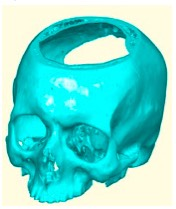% VLFLofVMdelaunay(V,vs,s) - returns a surface model for a delaunay reconstructed volume model% (by Tim Lueth, VLFL-Lib, 2015-JAN-20 as class: VOXELS)% % See also: VLFLofVMdelaunay, VLFLofVMmarchcube% % [VL,FL,TR4]=VLFLofVMdelaunay(V,[vs,s])% === INPUT PARAMETERS ===% V: Voxel Model (~=0 is reconstruction)% vs: voxel size, optional% s: step size% === OUTPUT RESULTS ======% VL: Vertex list% FL: Facet list% TR4: Delaunay tetrahedron model%
###### TR2ofimage(I,t)- returns a Delaunay triangulation for a grayscale image% TR2ofimage(I,t) - returns a Delaunay triangulation for a grayscale image% (by Tim Lueth, VLFL-Lib, 2015-JAN-20 as class: VOXELS)% % Type = 0 => triangulation contains all pixels and pixel facets% Type = 1 => triangulation contains only triangulated boundary facets% % WARNING: This procedure contains a real Matlab coding bug: If the two % lines of procedure TR2reduce are directly inserted in the code, an % error occurs by the isInterior procedure that is not explainable.% (Status of: 2015-01-20)% % TR2=TR2ofimage(I,[t])% === INPUT PARAMETERS ===% I: Image nxm% t: type of triangulation (default is 1)% === OUTPUT RESULTS ======% TR2: delaunay triangulation% % EXAMPLE: Analyze dicom images:% subplot (1,2,1); TR2ofimage (I1>1400,0); % subplot (1,2,2); TR2ofimage (I1>1400,1); %
###### TR3ofVM(V,vs,s)- returns a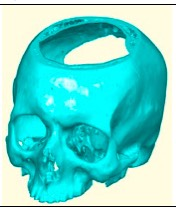% TR3ofVM(V,vs,s) - returns a% (by Tim Lueth, VLFL-Lib, 2015-JAN-20 as class: VOXELS)% % See also: VLFLofVMdelaunay, VLFLofVMmarchcube% % [TR4,TR3]=TR3ofVM(V,[vs,s])% === INPUT PARAMETERS ===% V: % vs: % s: % === OUTPUT RESULTS ======% TR4: % TR3: %
###### VM(I)- returns a matrix of reduced size if required (squeeze)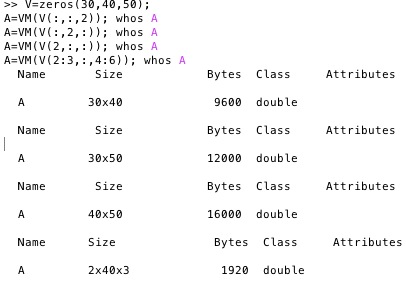% VM(I) - returns a matrix of reduced size if required (squeeze)% (by Tim Lueth, VLFL-Lib, 2015-JAN-19 as class: VOXELS)% % Obsolete fnctn: Better use Matlab fnctn squeeze directly% This is an auxiliary fnctn to take out a nxnxm submatrix from a nxnxm % matrix, since Matlab does not treat nxnxm matrice orthogonal to nxm % matrices. This fnctn is preferable to take out an orthogonal slice or % subvolume from a voxel model. (Status of: 2017-02-16)% % See also: VMimage, VMimrot90, VMmontage, VMplot, VMpseudo3D, % VMreaddicom, VMreaddicomdir, VMuidicom% % I=VM(I)% === INPUT PARAMETERS ===% I: Matrix n x n x m% === OUTPUT RESULTS ======% I: Matrix n x n x m% % EXAMPLE: Different subvolums from a 3D voxel model:% V=zeros(30,40,50);% A=VM(V(:,:,2)); whos A% A=VM(V(:,2,:)); whos A% A=VM(V(2,:,:)); whos A% A=VM(V(2:3,:,4:6)); whos A%
###### VLofVM(VM,vs)- returns the voxel coordinates as point list (can be long)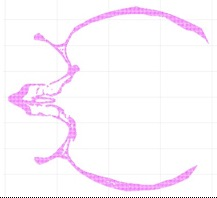% VLofVM(VM,vs) - returns the voxel coordinates as point list (can be long)% (by Tim Lueth, VLFL-Lib, 2015-JAN-14 as class: VOXELS)% % % VL=VLofVM(V>0.5); returns all voxel coordinates of V(:,:,:)>0.5% I=V(V>0.5); contains all voxel intensities for the VL% VL=VLofVM(V>0.5,[0.4 0.4 1]) returns all cartesian coordinates using vs% (Status of: 2017-03-20)% % See also: VLplot, VLscatter, VLofimage% % VL=VLofVM(VM,[vs])% === INPUT PARAMETERS ===% VM: Voxel model (mxmxn) or image stack% vs: optional voxel size% === OUTPUT RESULTS ======% VL: Vertex list% % EXAMPLE: VL=VLofVM(V(:,:,64)>1400); VLplot(VL,'m.')% [VL,EL,FL]=VLofimage(:,:,64)>1200, 1 , 2);% % [VL,C]=VLofVM(V(:,:,1:10)>1400); C=V(V(:,:,1:10)>1400);%
###### VMimage (IM,p)- returns three crosssectional views of an image stack (voxel model)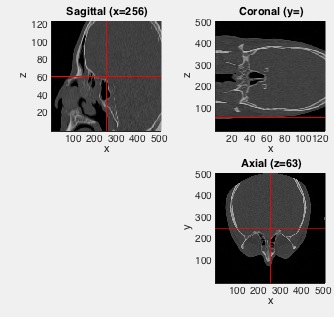% VMimage (IM,p) - returns three crosssectional views of an image stack (voxel model)% (by Tim Lueth, NAV-Lib, 2015-JAN-14 as class: VISUALIZATION)% % By default the center of the image is used to create the three % crosssectional views. (Status of: 2017-04-15)% % Introduced first in SolidGeometry 2.1% % See also: VM, VMimrot90, VMmontage, VMplot, VMpseudo3D, VMreaddicom, % VMreaddicomdir, VMuidicom% % VMimage(IM,[p])% === INPUT PARAMETERS ===% IM: 3D Matrix % p: optional point of interest (row, column, slice) % % See also: VM, VMimrot90, VMmontage, VMplot, VMpseudo3D, VMreaddicom, % VMreaddicomdir, VMuidicom% % % Copyright 2015-2017 Tim C. Lueth
###### VMimrot90(I)- returns an 90 degree rotated image (nxm) or image stack (nxmxk)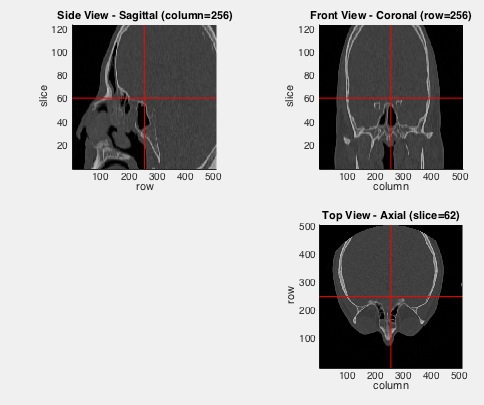% VMimrot90(I) - returns an 90 degree rotated image (nxm) or image stack (nxmxk)% (by Tim Lueth, VLFL-Lib, 2015-JAN-13 as class: VOXELS)% % +90 degree: fliplr(I')% 180 degree: flipud(I)% -90 degree: flipud(I') (Status of: 2017-02-16)% % See also: VM, VMimage, VMmontage, VMplot, VMpseudo3D, VMreaddicom, % VMreaddicomdir, VMuidicom% % NI=VMimrot90(I)% === INPUT PARAMETERS ===% I: image or image stack% === OUTPUT RESULTS ======% NI: new image or image stack%
###### SGintersectFacetPoints(A,B)- calculates a list of crossing facet pairs and crossing points of 2 solids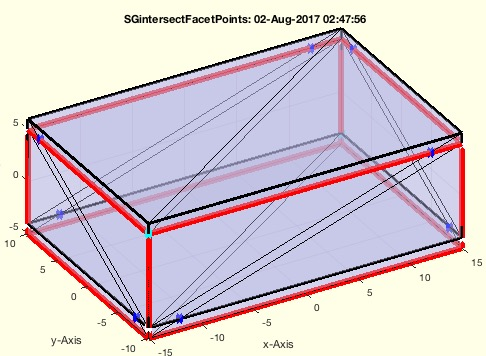% SGintersectFacetPoints(A,B) - calculates a list of crossing facet pairs and crossing points of 2 solids% (by Tim Lueth, VLFL-Lib, 2015-JAN-12 as class: SURFACES)% % This fnctn is now (2017-Aug) 5 times faster than the fastest % MatlabCentral implementation mesh2mesh. Therefor fnctn VLcrossingSG % should be based on SGintersectFacetPoints in future for collision % checks.% This fnctn is an elementary part of SGbool1-SGbool3 and was isolated % and improved for SGbool4 in 2017-07-31.% The 6th row is may be obsolete, since it is not used in % VLFLinsertFacetPoints% The resulting format of NPL is:% col 01: facet of A% col 02: facet of B% col 03-05: crossing point% col 06: intersection information % col 06: sign is inside/out 1..3 is % cal 06: value ==> 1..3 means 1st facet egde; 0.1..0.3 means 2nd face % edge% (Status of: 2017-08-03)% % Introduced first in SolidGeometry 2.1% % See also: crossingfacets2VLFL, cross2F, intersectstriangle, % VLFLinsertFacetPoints% % [NPL,IEL]=SGintersectFacetPoints(A,B)% === INPUT PARAMETERS ===% A: Solid A% B: Solid B% === OUTPUT RESULTS ======% NPL: New point list% IEL: edge list % % EXAMPLE: % A=SGbox([30,20,10]); B=SGtransP(A,[1 2 3]);% SGintersectFacetPoints(A,B)% % % See also: crossingfacets2VLFL, cross2F, intersectstriangle, % VLFLinsertFacetPoints% % % Copyright 2015-2017 Tim C. Lueth
###### VLFLinsertFacetPoints(VLA,FLA,NPL)- This function retesselates all surfaces of a solid by inserting points into existing facets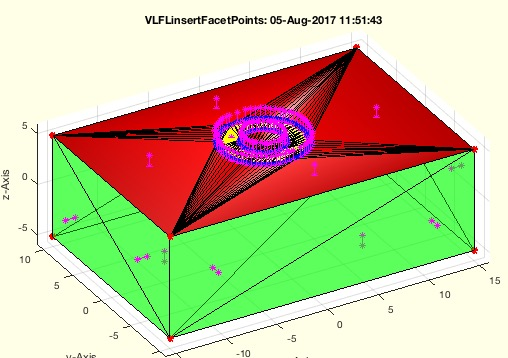% VLFLinsertFacetPoints(VLA,FLA,NPL) - This fnctn retesselates all surfaces of a solid by inserting points into existing facets% (by Tim Lueth, VLFL-Lib, 2015-JAN-12 as class: SURFACES)% % This fnctn is an elementary part of SGbool1-SGbool3 and was isolated % for SGbool4 in 2017-08-02% It is not enough just to add the points to a triangle but also it is % necessary to add edges inside of the facet for each cutting edge! % Otherwise it is not possible to reconnect the two separated handled % Solids!% The final result are THREE retesselate facet list: NFL and SFL and UFL% The orientation of the faces of SFL are changed. (Status of: 2017-08-06)% % Introduced first in SolidGeometry 2.1% % See also: SGintersectFacetPoints, crossingfacets2VLFL, cross2F, % intersectstriangle% % [XVL,XFIL,NFL,SFL,UFL]=VLFLinsertFacetPoints(VLA,FLA,NPL)% === INPUT PARAMETERS ===% VLA: Solid A% FLA: Solid B% NPL: New Point List created by SGintersectFacetPoints% === OUTPUT RESULTS ======% XVL: Original point list plus additional points% XFIL: Facet index list of original facets that were changed% NFL: Facets that belong completely to crossing points (red)% SFL: Facets that connect untouched points and crossing points (yellow)% UFL: Facets that are not touched (green)% % EXAMPLE: % A=SGbox([30,20,10]); B=SGtransP(A,[1 2 3]); % SGintersectFacetPoints(A,B); NPL=ans% A=SGbox([30,20,10]); B=SGofCPLz([PLcircle(4);NaN % NaN;PLcircle(2)],10);SGintersectFacetPoints(A,B); NPL=ans% VLFLinsertFacetPoints(A.VL,A.FL,NPL)% VLFLinsertFacetPoints(B.VL,B.FL,NPL(:,[2 1 3:end]))% % See also: SGintersectFacetPoints, crossingfacets2VLFL, cross2F, % intersectstriangle% % % Copyright 2015-2017 Tim C. Lueth
###### exp_2015_01_10c (bo)-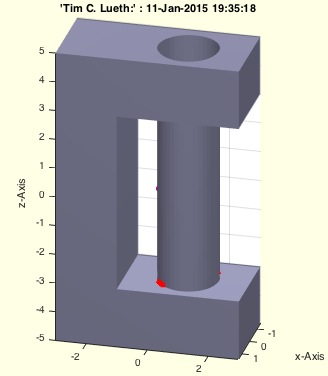% exp_2015_01_10c (bo) - % (by Tim Lueth, VLFL-Lib, 2015-JAN-11 as class: EXPERIMENTS)% % exp_2015_01_10c([bo])% === INPUT PARAMETERS ===% bo: %
###### SGbool3(flag,A,B)- returns the result of a boolean operation on two solids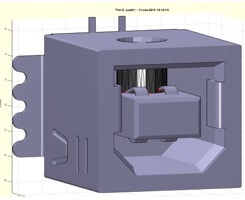% SGbool3(flag,A,B) - returns the result of a boolean operation on two % solids% (by Tim Lueth, VLFL-Lib, 2015-JAN-11 as class: SURFACES)% % First version that works completely correct. Next step is % retesselation. IgtG% A= A without B% B= B without A% += A combined with B% x= A intersected with B% There is one problem with concave surfaces (Status of: 2015-01-11)% % SGX=SGbool3(flag,A,B)% === INPUT PARAMETERS ===% flag: Boolean operator ('AB+x')% A: Solid A (VL/FL)% B: Solid B (VL/FL)% === OUTPUT RESULTS ======% SGX: resulting surface geometry%
###### exp_2015_01_11 (SGA,bo)- EXPRIMENT TO drill through STL-FIles using SGbool% exp_2015_01_11 (SGA,bo) - EXPRIMENT TO drill through STL-FIles using SGbool% (by Tim Lueth, VLFL-Lib, 2015-JAN-11 as class: EXPERIMENTS)% % exp_2015_01_11(SGA,[bo])% === INPUT PARAMETERS ===% SGA: Solid Geoemtry% bo: %
###### FLorder(FL)- returns a shifted and ordered facet list% FLorder(FL) - returns a shifted and ordered facet list% (by Tim Lueth, VLFL-Lib, 2015-JAN-11 as class: AUXILIARY PROCEDURES)% % The facet list is rotated line by line so that the smallest vertex % index is in the left column. % % In contrast to FLshift, by using FLorder the facet list is sorted by % increasing numbers of column sortrows(FL,[1 2 3]). (Status of: % 2017-02-21)% % See also: ELorder, FLseparate, TRorder, SGorder, SGseparate% % FL=FLorder(FL)% === INPUT PARAMETERS ===% FL: Original facet list% === OUTPUT RESULTS ======% FL: Final facet list% % EXAMPLE: % FL=[10 20 30; 30 10 20; 20 30 10] % FL=[FL*1.5;FL]% FLorder(FL)%
###### exp_2015_01_11 (SGA,bo)- EXPERIMENT that shows the power of SGboo3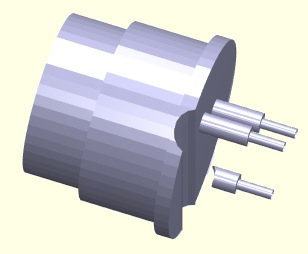% exp_2015_01_11 (SGA,bo) - EXPERIMENT that shows the power of SGboo3% (by Tim Lueth, VLFL-Lib, 2015-JAN-11 as class: EXPERIMENTS)% % exp_2015_01_11(SGA,[bo])% === INPUT PARAMETERS ===% SGA: Solid Geometry% bo: boolean operator%
###### exp_2015_01_10 (bo)- EXPERIMENT to the first usable function SGbool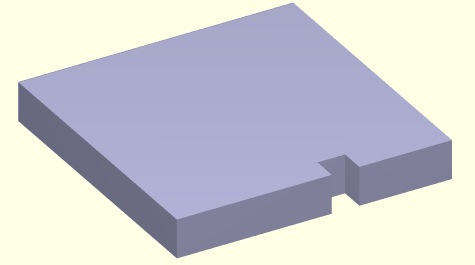% exp_2015_01_10 (bo) - EXPERIMENT to the first usable fnctn SGbool% (by Tim Lueth, VLFL-Lib, 2015-JAN-10 as class: EXPERIMENTS)% % exp_2015_01_10([bo])% === INPUT PARAMETERS ===% bo: flag; default is x (intersection)%
###### exp_2015_01_08m- EXPERIMENT that tesselates a solid as preparation for cutting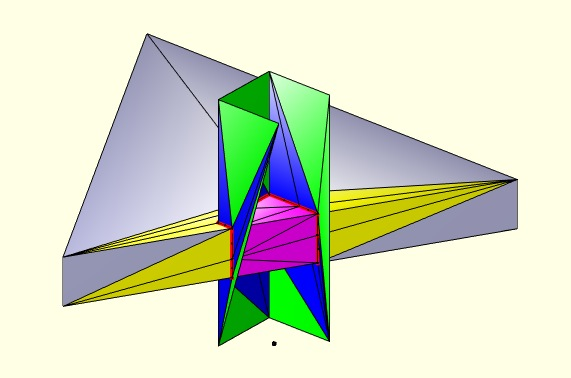% exp_2015_01_08m - EXPERIMENT that tesselates a solid as preparation for % cutting% (by Tim Lueth, VLFL-Lib, 2015-JAN-10 as class: SURFACES)% % NPL=exp_2015_01_08m% === OUTPUT RESULTS ======% NPL: %
###### exp_2015_01_08i- EXPERIMENT that tesselates a solid as preparation for cutting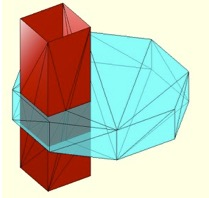% exp_2015_01_08i - EXPERIMENT that tesselates a solid as preparation for % cutting% (by Tim Lueth, VLFL-Lib, 2015-JAN-10 as class: SURFACES)% % exp_2015_01_08i%
###### SGisInterior(SG,VL)- returns the isInside Flag for a SG and a VL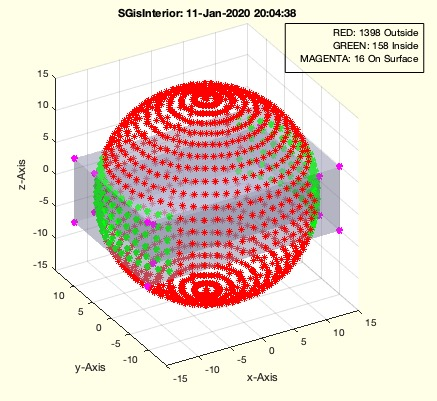% SGisInterior(SG,VL) - returns the isInside Flag for a SG and a VL% (by MATLAB-CENTRAL, VLFL-Lib, 2015-JAN-10 as class: SURFACES)% % Fast checking fnctn for points that are not on the surface. Surface % points belong to the inner points.% Bugs appear if points are at the same position but different z axis. % Could easily be solved.% This fnctn still has some bugs with vertices on the surface of the % solid. See mesh2mesh to learn how to improve VLFLinpolyhedron (Status % of: 2017-01-02)% % See also: mesh2mesh, VLFLinpolyhedron, BBiscollofVL, outboundingbox, % VLcrossingSG, crossingfacets2VLFL% % VIL=SGisInterior(SG,VL)% === INPUT PARAMETERS ===% SG: Solid Geoemtry% VL: Vertex list to test% === OUTPUT RESULTS ======% VIL: Vertex index list% % EXAMPLE: Test the probleatic surface points if this fnctn:% A=SGbox([30,20,10]); % VLcrossingSG(A,SGtrans(A,rotdeg(90))); % VL=VLcrossingSG(A,SGtrans(A,rotdeg(90)));% VL=unique(VL,'rows');% SGisInterior(A,VL)% % More sucessfull test% A=SGbox([30,20,10]); SGisInterior(A,20*(rand(100,3)-0.5));%
###### VLFLfaceNormal(VL,FL,fi,th)- returns the normal vector of the facet list and cutted (1e-4) length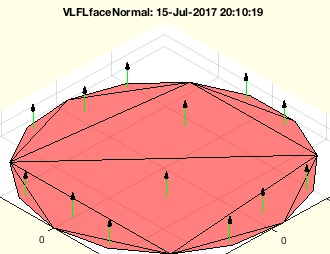% VLFLfaceNormal(VL,FL,fi,th) - returns the normal vector of the facet list and cutted (1e-4) length% (by Tim Lueth, VLFL-Lib, 2015-JAN-09 as class: ANALYTICAL GEOMETRY)% % This is a fnctn to adapt the VLFL syntax to R2014b.% The matlab cross-fnctn of triangualtion results returns calucation % error in size (1e-4) (Status of: 2017-08-14)% % Introduced first in SolidGeometry 2.1% % See also: VLFLvertexNormal, PLnorm, PLELnorm, PLFLfaceNormal, VLnorm, % VLFLnormf, VLedgeNormal, VLFLfaceAngles% % [NL,AL]=VLFLfaceNormal(VL,FL,[fi,th])% === INPUT PARAMETERS ===% VL: Vertex list n x 3% FL: Facet list% fi: optional value; facet index (list)% th: % === OUTPUT RESULTS ======% NL: Normal vector list% AL: Area list; % % EXAMPLE: % [VL,FL]=PLFLofCPLdelaunay(PLcircle(1)); VL=VLaddz(VL);% VLFLfaceNormal(VL,FL)% % See also: VLFLvertexNormal, PLnorm, PLELnorm, PLFLfaceNormal, VLnorm, % VLFLnormf, VLedgeNormal, VLFLfaceAngles% % % Copyright 2015-2018 Tim C. Lueth
###### exp_2015_01_08b- EXPERIMENT SCRIPT for testing crossingfacets2VLFL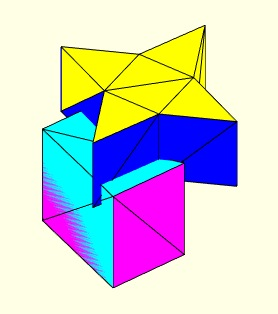% exp_2015_01_08b - EXPERIMENT SCRIPT for testing crossingfacets2VLFL% (by Tim Lueth, VLFL-Lib, 2015-JAN-08 as class: EXPERIMENTS)% % exp_2015_01_08b%
###### SGcut(SG,z)- Cuts a solid geometry into 2 parts at a defined z-plane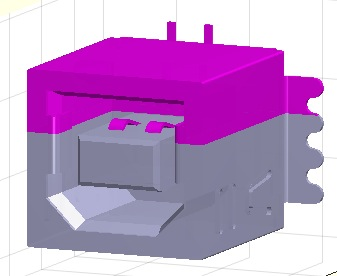% SGcut(SG,z) - Cuts a solid geometry into 2 parts at a defined z-plane% (by Tim Lueth, VLFL-Lib, 2015-JAN-08 as class: SURFACES)% % returns two solid solids (VL,FL). It is a good programming example how % to use SGslicer.% Without output parameter is shows the cutted parts using VLFLfigure% (Status of: 2017-01-29)% % Introduced first in SolidGeometry 2.1% % See also: SGcut, SGcut2, SGcutBB, SGcutT% % [SGA,SGB]=SGcut(SG,z)% === INPUT PARAMETERS ===% SG: Solid Geometry (VL,FL)% z: z value for slicing or interval [z1 z2]% === OUTPUT RESULTS ======% SGA: Solid below cutting plane (and above z2) (grey)% SGB: Solid above cutting plane (and in between z2) (magenta)% % EXAMPLE: SGcut (SGsample(7),+10);% % See also: SGcut, SGcut2, SGcutBB, SGcutT% % % Copyright 2015-2018 Tim C. Lueth
###### exp_2015_01_08- EXPERIMENT SCRIPT for testing SGcut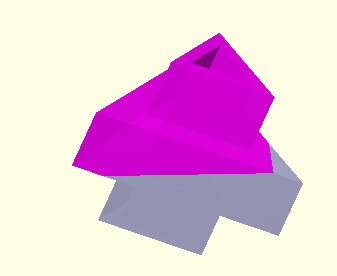% exp_2015_01_08 - EXPERIMENT SCRIPT for testing SGcut% (by Tim Lueth, VLFL-Lib, 2015-JAN-08 as class: EXPERIMENTS)% % exp_2015_01_08%
###### SGslicer(SG,z)- returns the delaunayTriangulation of the sliced plane% SGslicer(SG,z) - returns the delaunayTriangulation of the sliced plane% (by Tim Lueth, VLFL-Lib, 2015-JAN-07 as class: SLICES)% % Efficient, modern and powerful version of VLELslicer/VLELslicer2 that % uses delaunayTriangulation for reconstruction and calulation of upper % and lower cutted facets in addition.% Without output parameters, the result is shown in VLFLfigure% Modified in June 2015 to support already cutted objects (lines in the % cutting plane). There is still no possibility to cut parts at the outer % boundaries (surfaces) of the object. (Status of: 2015-06-21)% % [TR2,EL,SIL,FIL,FLO,FLU,warn]=SGslicer(SG,z)% === INPUT PARAMETERS ===% SG: Solid Geometry (VL,FL)% z: z value for slicing% === OUTPUT RESULTS ======% TR2: Delaunay Triangulation of the cutting plane% EL: Border Edge List of TR2 (TR2.Constraints)% SIL: Surface Index List (Index of separated surface areas)% FIL: Facet index list of the cutted facets -1=below, +1= up, 0=cutted% FLO: Facet list upper related to [SG.VL; TR2.Points]% FLU: Facet list lower related to [SG.VL; TR2.Points]% warn: % % EXAMPLE: SGslicer (SGsample(7),+10);%
###### exp_2015_01_07b- EXPERIMENT SCRIPT for testing SGslicer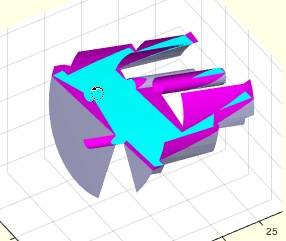% exp_2015_01_07b - EXPERIMENT SCRIPT for testing SGslicer% (by Tim Lueth, VLFL-Lib, 2015-JAN-07 as class: EXPERIMENTS)% % exp_2015_01_07b%
###### PLELhsort(PL,EL,SIL)- returns a hierarchy index list for a PL,EL,SIL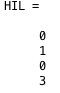% PLELhsort(PL,EL,SIL) - returns a hierarchy index list for a PL,EL,SIL% (by Tim Lueth, VLFL-Lib, 2015-JAN-05 as class: SLICES)% % PL,EL, and SIL are unchanged. The hierarchy index list contains the % index of the enclosing contour% With PLELhsort it is possible to find the outside contours of a slice % (0), which is sometimes better than a freeBoundary% (Status of: 2015-01-05)% % HIL=PLELhsort(PL,EL,SIL)% === INPUT PARAMETERS ===% PL: Point List% EL: Edge List% SIL: Surface Index List% === OUTPUT RESULTS ======% HIL: hierarchy index list%
###### exp_2015_01_05 (n,dz)- EXPERIMENT for hierarchical ordering of VLEL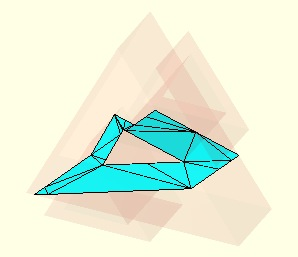% exp_2015_01_05 (n,dz) - EXPERIMENT for hierarchical ordering of VLEL% (by Tim Lueth, VLFL-Lib, 2015-JAN-05 as class: EXPERIMENTS)% % Testing procedure for SGslicer and ELofFLborder2 and PLELhsort% 1. Slicing using SGslicer% 2. Connect the border edges of the Triagulation using ELofFLborder2% 3. Calculate the Hierarchy index% 4. Use only the outer contours (Status of: 2015-01-05)% % exp_2015_01_05([n,dz])% === INPUT PARAMETERS ===% n: SGsample(positive); CPLsample(negative) or SG% dz: slicing step (positive) slice value (negative)%
###### colofn(i,colbar,colstr)- returns a color char for a number 0..7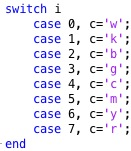% colofn(i,colbar,colstr) - returns a color char for a number 0..7% (by Tim Lueth, VLFL-Lib, 2015-JAN-05 as class: AUXILIARY PROCEDURES)% % simple auxiliary fnctn for color use in for loops (Status of: % 2017-06-17)% % See also: nofcolmap, color, VLcol% % c=colofn(i,[colbar,colstr])% === INPUT PARAMETERS ===% i: integer number% colbar: order of colors; default is 'kbgcmyrw'% colstr: colorstring such as 'R*--'% === OUTPUT RESULTS ======% c: color char string%
###### ELofFLborder2(FL)- returns the border edges of a surface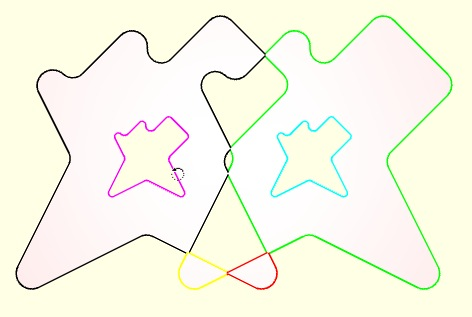% ELofFLborder2(FL) - returns the border edges of a surface% (by Tim Lueth, VLFL-Lib, 2015-JAN-05 as class: SLICES)% % First the surface is separated into areas that have no common edges % (ELorder). Then for each area, the edges with only one direction are % the border edges.% Should replace ELofFLborder one day. (Status of: 2015-01-05)% % [EL,SIL]=ELofFLborder2(FL)% === INPUT PARAMETERS ===% FL: Facet list% === OUTPUT RESULTS ======% EL: Edge list% SIL: Surface index list%
###### exp_2015_01_04b (SG,dz)- EXPERIMENT that shows the use of SGslicer and freeboundary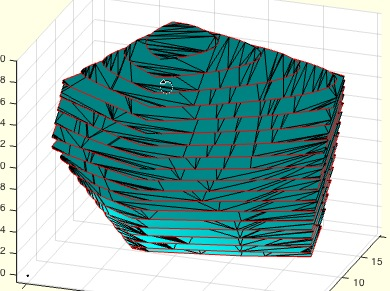% exp_2015_01_04b (SG,dz) - EXPERIMENT that shows the use of SGslicer and % freeboundary% (by Tim Lueth, VLFL-Lib, 2015-JAN-04 as class: EXPERIMENTS)% % Towards a reimplementation of VLFLclosure - here we see the sliced % freeboundary% If the procedure is interrupted by Ctrl-C you see the inner part of the % sliced solid (Status of: 2015-01-04)% % exp_2015_01_04b(SG,[dz])% === INPUT PARAMETERS ===% SG: Solid Geometry (VL,FL)% dz: slicing distance%
###### exp_2015_01_04 (SG,dz)- EXPERIMENT that shows the use of SGslicer and freeboundary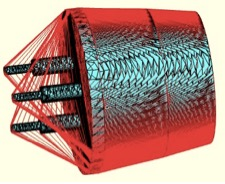% exp_2015_01_04 (SG,dz) - EXPERIMENT that shows the use of SGslicer and % freeboundary% (by Tim Lueth, VLFL-Lib, 2015-JAN-04 as class: EXPERIMENTS)% % Towards a reimplementation of VLFLclosure% If the procedure is interrupted by Ctrl-C you see the inner part of the % sliced solid (Status of: 2015-01-04)% % exp_2015_01_04(SG,[dz])% === INPUT PARAMETERS ===% SG: Solid Geometry (VL,FL)% dz: slicing distance%
###### SGslicer(SG,z)- returns the delaunayTriangulation of the sliced plane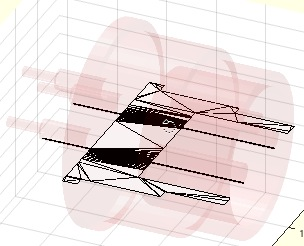% SGslicer(SG,z) - returns the delaunayTriangulation of the sliced plane% (by Tim Lueth, VLFL-Lib, 2015-JAN-04 as class: SLICES)% % More modern version of VLELslicer/VLELslicer2 that uses % delaunayTriangulation for reconstruction.% Without an output parameter, the result is shown y VLFLfigure (Status % of: 2015-01-04)% % [TR2,EL,SIL]=SGslicer(SG,z)% === INPUT PARAMETERS ===% SG: Solid Geometry (VL,FL)% z: z value for slicing% === OUTPUT RESULTS ======% TR2: Crossing vertex list of the selected slice% EL: Crossing line list of the selected slice% SIL: Normal vector list of the crossing lines% % EXAMPLE: Generate a simple octagon (5x5x20)% [VL,FL]=VLFLcylinder(20,5/cos(pi/8),8-1,1); % [CVL,CLL,NL]=VLELslicer (VL,FL,0)% VLELplot (CVL,CLL,'r*-',NL);%
###### SGseparate(SG,i)- plots or returns sorted surfaces or one surface of a solid geometry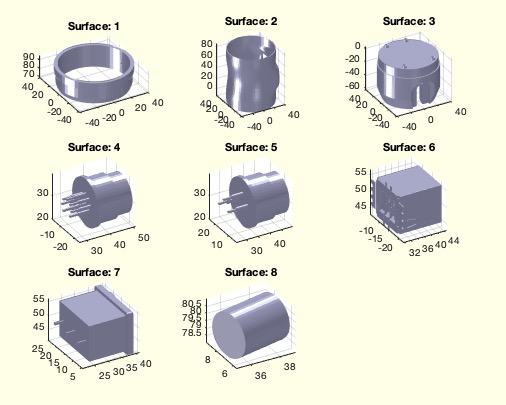% SGseparate(SG,i) - plots or returns sorted surfaces or one surface of a solid geometry% (by Tim Lueth, VLFL-Lib, 2015-JAN-04 as class: SURFACES)% % SGseparate is a newer (faster and more convinient) version of % VLFLseparate% Based on ELorder and VLELselect.% With only one surface is asked, surface is moved into 1st. Quadrant% With no output parmeter, the surface(s) are plotted using VLFLfigure% With no SG specified, VLFLui is used to read in an STL-File% 2017-06-14: i can be index or index list (Status of: 2017-07-11)% % Introduced first in SolidGeometry 2.1% % See also: SGsurfaces, SGanalyzeGroupParts, SGanalyzePenetration, % VLFLseparate, SGorder, TRorder% % [SG,SIL,n,BBi]=SGseparate([SG,i])% === INPUT PARAMETERS ===% SG: Solid Geometry; if empty% i: index list of single/selected surface% === OUTPUT RESULTS ======% SG: Solid Geoemtry% SIL: Surface Index List% n: number of closed surfaces% BBi: Cell list of Bounding Boxes of the Solids% % EXAMPLE: % SGseparate(J1,[1,2,4])% % See also: SGsurfaces, SGanalyzeGroupParts, SGanalyzePenetration, % VLFLseparate, SGorder, TRorder% % % Copyright 2015-2018 Tim C. Lueth
###### exp_2015_01_03b(n,a)- Experiment to improve TRofSG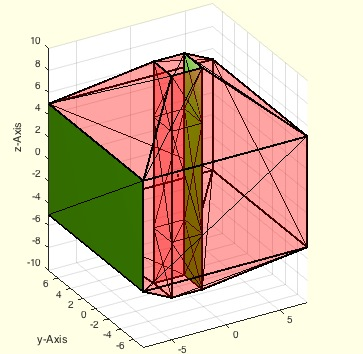% exp_2015_01_03b(n,a) - Experiment to improve TRofSG% (by Tim Lueth, VLFL-Lib, 2015-JAN-03 as class: EXPERIMENTS)% % [ELFL,SIL,nv]=exp_2015_01_03b([n,a])% === INPUT PARAMETERS ===% n: n in sGsample(positive) or CPLsample (negative)% a: index or normal vector% === OUTPUT RESULTS ======% ELFL: % SIL: % nv: %
###### exp_2015_01_03(n)- EXPERIMENT to remove the bug from PLELofFeatureEdges2 in FLfeatureEdgeSurface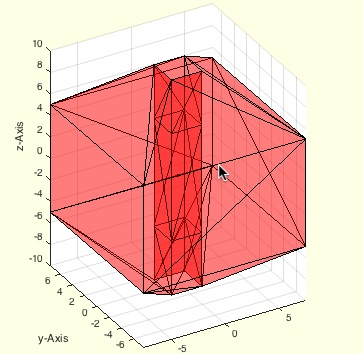% exp_2015_01_03(n) - EXPERIMENT to remove the bug from % PLELofFeatureEdges2% (by Tim Lueth, VLFL-Lib, 2015-JAN-03 as class: EXPERIMENTS)% % The BUG existing in Matlab is that % faceNormal NNED TO BE ROUNDED WITH 1e-6.% Afterwards the procedure works well. (Status of: 2015-01-03)% % ELFL=exp_2015_01_03([n])% === INPUT PARAMETERS ===% n: % === OUTPUT RESULTS ======% ELFL: %
###### TR3ofSG(SG)- returns the triangulation for a solid gemeotry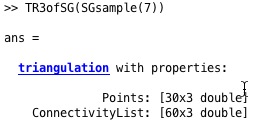% TR3ofSG(SG) - returns the triangulation for a solid gemeotry% (by Tim Lueth, VLFL-Lib, 2015-JAN-03 as class: SURFACES)% % single line macro: simple auxiliary procedure (Status of: 2015-01-03)% % TR=TR3ofSG(SG)% === INPUT PARAMETERS ===% SG: SG.VL, SG.FL% === OUTPUT RESULTS ======% TR: Triangulation nx3%
###### FEplot(A,)- plots the featureEdges of TR, SG or VLFL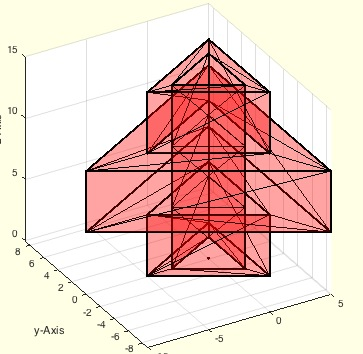% FEplot(A,) - plots the featureEdges of TR, SG or VLFL% (by Tim Lueth, VLFL-Lib, 2015-JAN-03 as class: VISUALIZATION)% % FEplot(SG)% FEplot(VL,FL)% default angle is 1e-3 (Status of: 2017-04-05)% % Introduced first in SolidGeometry 2.1% % See also: FSplot, FLfeatureEdgeSurface2, PLELofFeatureEdges, % PLELofFeatureEdges2, TRfeatureEdgeFacets, FLfeatureEdgeSurface% % FEplot(A,[])% === INPUT PARAMETERS ===% A: surface (Tetrahedron, Triangulation, Solid)% % EXAMPLE: FEplot(SGsample(3))% % See also: FSplot, FLfeatureEdgeSurface2, PLELofFeatureEdges, % PLELofFeatureEdges2, TRfeatureEdgeFacets, FLfeatureEdgeSurface% % % Copyright 2015-2017 Tim C. Lueth
###### PLradialEdges(PL,R,cvk,wmin,wmax,KL,m)- returns a point list (PL) with rounded edges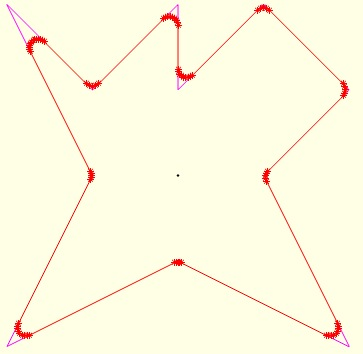% PLradialEdges(PL,R,cvk,wmin,wmax,KL,m) - returns a point list (PL) with rounded edges % (by Tim Lueth, VLFL-Lib, 2015-JAN-02 as class: ANALYZING PROCEDURES)% % PLradialEdges IS INDEPENDENT AND NOT USING PLtangentcirc!% % Replaces all/convex/concave edges with an angle in a defined intervall % [0,180] by a radial curve. The number of curve points can be specified % too (default is nofrd). The fnctns accepts a single CPL (without NaN) % or PL and returns always a PL! (Status of: 2018-07-28)% % Introduced first in SolidGeometry 2.1% % See also: VLradialEdges, VLtangentcirc, PLtangentcirc% % NPL=PLradialEdges(PL,[R,cvk,wmin,wmax,KL,m])% === INPUT PARAMETERS ===% PL: Point list nx2% R: Radius; default is 1% cvk: 0==all; +1=concave, -1=convex% wmin: minimal abs angle to handle; default is 0% wmax: maximum abs angle to handle; default is pi% KL: nr of sections of a line; 2 are required for radius at 90degree;% % m: number of points; default is nofrd% === OUTPUT RESULTS ======% NPL: New point list% % EXAMPLE: VLFLfigure; % C=CPLsample(1); % CPLplot(C,'m'); CPLplot(PLradialEdges(C,1));% PLradialEdges(PLstar(50,10),5);% % See also: VLradialEdges, VLtangentcirc, PLtangentcirc% % % Copyright 2015-2018 Tim C. Lueth
###### PLangle(PL)- returns the angles of a points list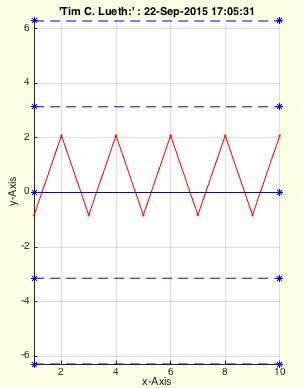% PLangle(PL) - returns the angles of a points list% (by Tim Lueth, VLFL-Lib, 2015-JAN-02 as class: ANALYZING PROCEDURES)% % In contrast to VLangle, PLangle uses crossz to calculate an % orientation: Positive values are mathematical positive (right hand). % (Status of: 2017-08-12)% % Introduced first in SolidGeometry 2.1% % See also: VLangle, diffangle, PLangle2% % [w,d]=PLangle(PL)% === INPUT PARAMETERS ===% PL: vector list% === OUTPUT RESULTS ======% w: angle list% d: distance list% % EXAMPLE: % [PLstar(10,10) PLangle(PLstar(10,10))/pi*180]% % See also: VLangle, diffangle, PLangle2% % % Copyright 2015-2017 Tim C. Lueth
###### exp_2015_01_02 (nr)- EXPERIMENT to show the use of FLfeatureEdgeSurface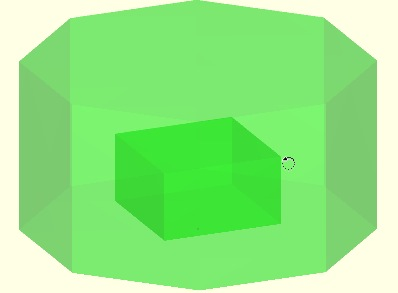% exp_2015_01_02 (nr) - EXPERIMENT to show the use of FLfeatureEdgeSurface% (by Tim Lueth, VLFL-Lib, 2015-JAN-02 as class: EXPERIMENTS)% % Simple experiment to test the procedure FLfeatureEdgeSurface, similar % to 2014_12_31 (Status of: 2015-01-02)% % exp_2015_01_02([nr])% === INPUT PARAMETERS ===% nr: Nr of SGsample (positive value) or CPLsample (negative value)% % EXAMPLE: exp_2015_01_02 (-7)%
###### TRofSGxor(SG,TR4)- removes tetrahedrons to create features edges which are existing in a surface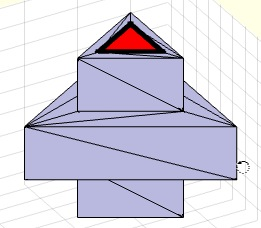% TRofSGxor(SG,TR4) - removes tetrahedrons to create features edges which % are existing in a surface% (by Tim Lueth, VLFL-Lib, 2015-JAN-02 as class: TETRAHEDRONS)% % This procedure calculates the freeBoundary of the points of a surface % model and compares the featureEdges of the freeBoundary with the % original featureEdges of a solid. All tetrahedrons are removed linked % to featureEdges that exist in the original surface but not in the % tetrahedron model.% An optional input argument is a preprocessed tetrahedron triangulation.% This procedure is used by TRofSG. In combination with TRofSGdiff % (removement of outside tetrahedons), TRofSGxor (removement of outside % tetrahedons) will create a tetrahedron triangulation for one single % surface triangulation. This procedure works for only ONE closed surface.% (Status of: 2015-01-02)% % TR4=TRofSGxor(SG,[TR4])% === INPUT PARAMETERS ===% SG: Solid geometry (SG.VL,SG>FL)% TR4: tetrahedron triangulation% === OUTPUT RESULTS ======% TR4: tetrahedron triangulation volume model%
###### TRofSGdiff(SG,TR4)- removes tetrahedrons that have features edges which are not existing in a surface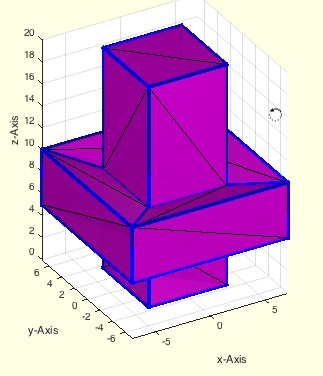% TRofSGdiff(SG,TR4) - removes tetrahedrons that have features edges % which are not existing in a surface% (by Tim Lueth, VLFL-Lib, 2015-JAN-02 as class: TETRAHEDRONS)% % This procedure calculates the freeBoundary of the points of a surface % model and compares the featureEdges of the freeBoundary with the % original featureEdges of a solid. All tetrahedrons are removed that % create featureEdges that are not existing in the original surface.% An optional input argument is a preprocessed tetrahedron triangulation.% This procedure is used by TRofSG. In combination with TRofSGxor % (removement of outside tetrahedons), TRofSGdiff (removement of outside % tetrahedons) will create a tetrahedron triangulation for one single % surface triangulation. This procedure works for only ONE closed surface.% (Status of: 2015-01-02)% % TR4=TRofSGdiff(SG,[TR4])% === INPUT PARAMETERS ===% SG: Solid geometry (SG.VL,SG>FL)% TR4: tetrahedron triangulation% === OUTPUT RESULTS ======% TR4: tetrahedron triangulation volume model%
###### PLstar(R,nf,dw,Ry,ow,sk)- returns a 2D point list of a star or star segment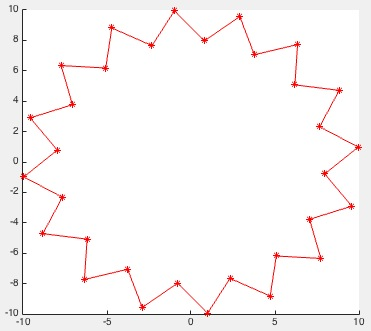% PLstar(R,nf,dw,Ry,ow,sk) - returns a 2D point list of a star or star segment% (by Tim Lueth, VLFL-Lib, 2015-JAN-01 as class: AUXILIARY PROCEDURES)% % PLstar starts not(!) at (R,0), but the first edge is going in % y-direction. This has been done to simply generate squares or % 2*n-Polygons. By using the offset angle, it is possible to start where % you want. (Status of: 2017-01-05)% % See also: PLcircle, PLcircseg, PLevolvente, PLgear, PLhelix, PLkidney, % PLrand, PLspiral, PLsquare% % PL=PLstar(R,[nf,dw,Ry,ow,sk])% === INPUT PARAMETERS ===% R: Radius% nf: number of facets (default is nofrd (R,0.05))% dw: circle segment ](0..2(pi] (default is 2*pi)% Ry: Radius if ellipse (default is R)% ow: Offset angle% sk: shortage factor;default 0.0% === OUTPUT RESULTS ======% PL: Point / Vertex list%
###### TRofSG(SG)- Creates a tetrahedron model from a surface model based on feature edges% TRofSG(SG) - Creates a tetrahedron model from a surface model based on % feature edges% (by Tim Lueth, VLFL-Lib, 2014-DEZ-31 as class: TETRAHEDRONS)% % extracted from exp_2014-12-30d. Feature edges that exist in the % freeBoundary of a delaunayTriangulation, created from the points of a % surface model, but exist not in the surface model, can be removed % recursively by removing the attached tetrahedrons. % WORK in progress% Use FEplot or exp_2014_12_30e to understand the reason for failing % (Status of: 2015-01-02)% % TR=TRofSG(SG)% === INPUT PARAMETERS ===% SG: Solid Surface Model% === OUTPUT RESULTS ======% TR: Solid Tetrahedron Model% % EXAMPLE: Create two tetrahedron volume models from two surfaces% VLFLfigure; view(-30,30); % TR=TRofSG(SGofCPLz(CPLsample(12),10)); TRplot(TR)%
###### FLfeatureEdgeSurface(TR3,angle)- returns a surface facet list based on a given surface triangulation% FLfeatureEdgeSurface(TR3,angle) - returns a surface facet list based on a given surface triangulation% (by Tim Lueth, VLFL-Lib, 2014-DEZ-30 as class: SURFACES)% % This fnctn analyses the feature edges of a surface, reconnects them % into contours, and uses delaunay triangulation on the same points to % recalculate the surface. It uses % TRfeatureEdgeFacets% PLELofFeatureEdges2% FLofVLELn % for reconstruction. (Status of: 2017-04-05)% % See also: FLfeatureEdgeSurface, FLfeatureEdgeSurface2, % PLELofFeatureEdges, PLELofFeatureEdges2, TRfeatureEdgeFacets, FEplot% % FL=FLfeatureEdgeSurface(TR3,angle)% === INPUT PARAMETERS ===% TR3: Surface volume triangulation% angle: filter angle for feature edges% === OUTPUT RESULTS ======% FL: Facet List w.r.t TR3.Points% % EXAMPLE: Create a solid and recreate the surface% SG=SGsample(7);% VLFLplots(SG.VL, % FLfeatureEdgeSurface(triangulation(SG.FL,SG.VL),0.01),'g');% %
###### FLofVLELn(VL,EL,nv)- returns the facet list for a vertex list and a planar edge list in 3D% FLofVLELn(VL,EL,nv) - returns the facet list for a vertex list and a % planar edge list in 3D% (by Tim Lueth, VLFL-Lib, 2014-DEZ-30 as class: AUXILIARY PROCEDURES)% % Similiar to FLofVLEL, using delaunayTri, bit in addition the contour is % decomposed in facet with same normal vectors. The result is much better % than FLofVLEL/Delaunaytri, if enough vertices are used.% Use FLofVLEL2 (VL,EL) for optimal results% Use FLofVLEL (VL,EL) for delaunay results% Use FLofVL for quick and dirty results% It is used in combination with feature edges.% (Status of: 2012-11-17)% % FL=FLofVLELn(VL,EL,nv)% === INPUT PARAMETERS ===% VL: Vertex list% EL: Edge list% nv: normal vector, has to be correct% === OUTPUT RESULTS ======% FL: Facet list%
###### PLELofFeatureEdges2(TR,alpha)- returns sorted closed polygon edge lists of the feature edges of a solid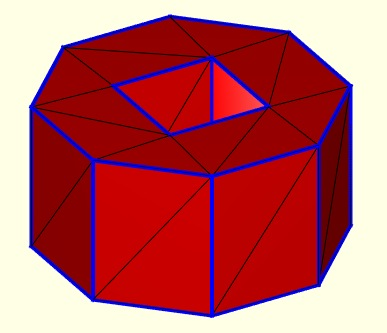% PLELofFeatureEdges2(TR,alpha) - returns sorted closed polygon edge lists of the feature edges of a solid% (by Tim Lueth, VLFL-Lib, 2014-DEZ-30 as class: SURFACES)% % fast and powerful fnctn that links the feature edges to closed polygon % edge lists with a defined normal vector% Uses: TRfeatureEdgeFacets% In contrast to PLELofFeatureEdges2, an additional column is added that % identifies the planes (Status of: 2017-04-05)% % See also: FLfeatureEdgeSurface, FLfeatureEdgeSurface2, % PLELofFeatureEdges, TRfeatureEdgeFacets, FEplot% % [ELFL,SIL]=PLELofFeatureEdges2(TR,[alpha])% === INPUT PARAMETERS ===% TR: surface triangulation for feature edges% alpha: feature edge angle% === OUTPUT RESULTS ======% ELFL: % SIL: Selected Index List for % % EXAMPLE: Recreate the polygons of a % closeall; SG=SGsample(16); % TR=triangulation(SG.FL,SG.VL); % VLFLfigure; [ELFL,SIL]=PLELofFeatureEdges (TR);%
###### exp_2014_12_31 (nr)- EXPERIMENT to reconstruct the surface% exp_2014_12_31 (nr) - EXPERIMENT to reconstruct the surface % (by Tim Lueth, VLFL-Lib, 2014-DEZ-30 as class: EXPERIMENTS)% % exp_2014_12_31([nr])% === INPUT PARAMETERS ===% nr: SGsample number or CPLsample number%
###### exp_2014_12_30- EXPERIMENT to develop the function TRofSG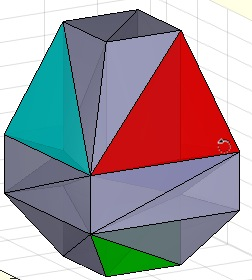% exp_2014_12_30 - EXPERIMENT to develop the fnctn TRofSG% (by Tim Lueth, VLFL-Lib, 2014-DEZ-30 as class: EXPERIMENTS)% % There exist several experiments of this day% exp_2014_12_30: showing the freeBoundary of delaunayTriangulation% exp_2014_12_30b: showing the additional featureEdges% exp_2014_12_30c: development of TRofSGdiff% exp_2014_12_30d: core part of TRofSGdiff% exp_2014_12_30e: showing the method of TRofSGxor% exp_2014_12_30f: show the final use of TRofSG% (Status of: 2015-01-02)% % exp_2014_12_30% % EXAMPLE: Testing TRofSG;% exp_2014_12_30f(7)%
###### exp_2014_12_29(nr)-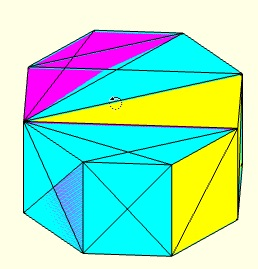% exp_2014_12_29(nr) - % (by Tim Lueth, VLFL-Lib, 2014-DEZ-29 as class: EXPERIMENTS)% % NL=exp_2014_12_29([nr])% === INPUT PARAMETERS ===% nr: % === OUTPUT RESULTS ======% NL: %
###### exp_2014_12_26b(n)- EXPERIMENT extruding different CPL analyzing inside & outside points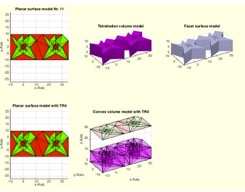% exp_2014_12_26b(n) - EXPERIMENT extruding different CPL analyzing % inside & outside points% (by Tim Lueth, VLFL-Lib, 2014-DEZ-26 as class: EXPERIMENTS)% % procedure 2014_12_26 generates CPLs using CPLsample.% In comparison to 2014_12_26, this procedure also analyzes the % individual contours of the inside facets to compare the use of % tetrahedrons that consists only of points of inside or only of points % of outside. In SGsample(9) we see that the surface points of inner % surfaces are also used for outside surfaces. So, it is not unique to % extract tetrahedrons just from the points list% THE STILL EXISTING PROBLEM CAN BE SEEN WITH CPLsample(8) AND % CPLsample(9): (Status of: 2014-12-27)% % fa=exp_2014_12_26b([n])% === INPUT PARAMETERS ===% n: n for CPLsample% === OUTPUT RESULTS ======% fa: %
###### exp_2014_12_26 (n)- EXPERIMENT extruding different CPL analyzing outside points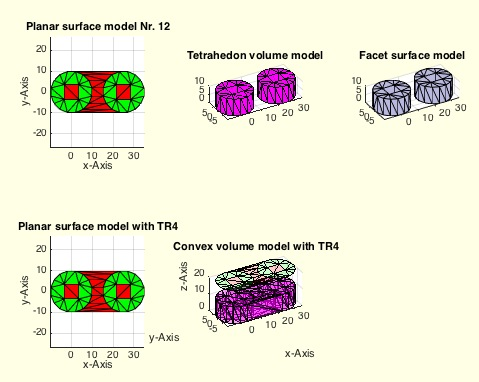% exp_2014_12_26 (n) - EXPERIMENT extruding different CPL analyzing % outside points% (by Tim Lueth, VLFL-Lib, 2014-DEZ-26 as class: EXPERIMENTS)% % procedure 2014_12_26 generates CPLs using CPLsample.% In comparison to 2014_12_26b, this procedure analyzes the individual % contours of the inside facets to compare the use of tetrahedrons that % consists only of points of outside. In SGsample(9) we see that the % surface points of inner surfaces are also used for outside surfaces. % So, it is not unique to extract tetrahedrons just from the points list% THE STILL EXISTING PROBLEM CAN BE SEEN WITH CPLsample(8) AND % CPLsample(9): (Status of: 2014-12-26)% % exp_2014_12_26([n])% === INPUT PARAMETERS ===% n: n for CPLsample%
###### CPLsample(Nr,T)- returns a a closed polygon list for different tests and experiments% CPLsample(Nr,T) - returns a a closed polygon list for different tests and experiments% (by Tim Lueth, VLFL-Lib, 2014-DEZ-26 as class: CLOSED POLYGON LISTS)% % Calling without an output parameter will always show the polygons% Calling without an input parameter will show all sample polygons% CPLsample can be called recursively to show the effect of fnctns used % on CPLsample-contours% Use CPLorder afterwards to order them. % Use CPLunite/CPLrecontour to process them. (Status of: 2018-07-28)% % Introduced first in SolidGeometry 2.1% % See also: CPLoftext, SGsample, VLsample, PLsample, VLFLsample, % CSGsample, SGerrorsample, testfunctTL, permutevector% % [CPL,TR3]=CPLsample([Nr,T])% === INPUT PARAMETERS ===% Nr: Number of sample closed polygon list or specific CPL for display% T: Parameter for SGtrans (Value, Vector, Matrix)% === OUTPUT RESULTS ======% CPL: Closed Polygon List% TR3: Optional delaunay triangulation of CPL using PLELofCPL% % EXAMPLE: Recontour overlapping contours% CPLsample(16)% CPLsample(CPLrecontour(CPLsample(16)))% CPLsample(CPLrecontour(flip(CPLsample(16))))% % See also: CPLoftext, SGsample, VLsample, PLsample, VLFLsample, % CSGsample, SGerrorsample, testfunctTL, permutevector% % % Copyright 2014-2018 Tim C. Lueth